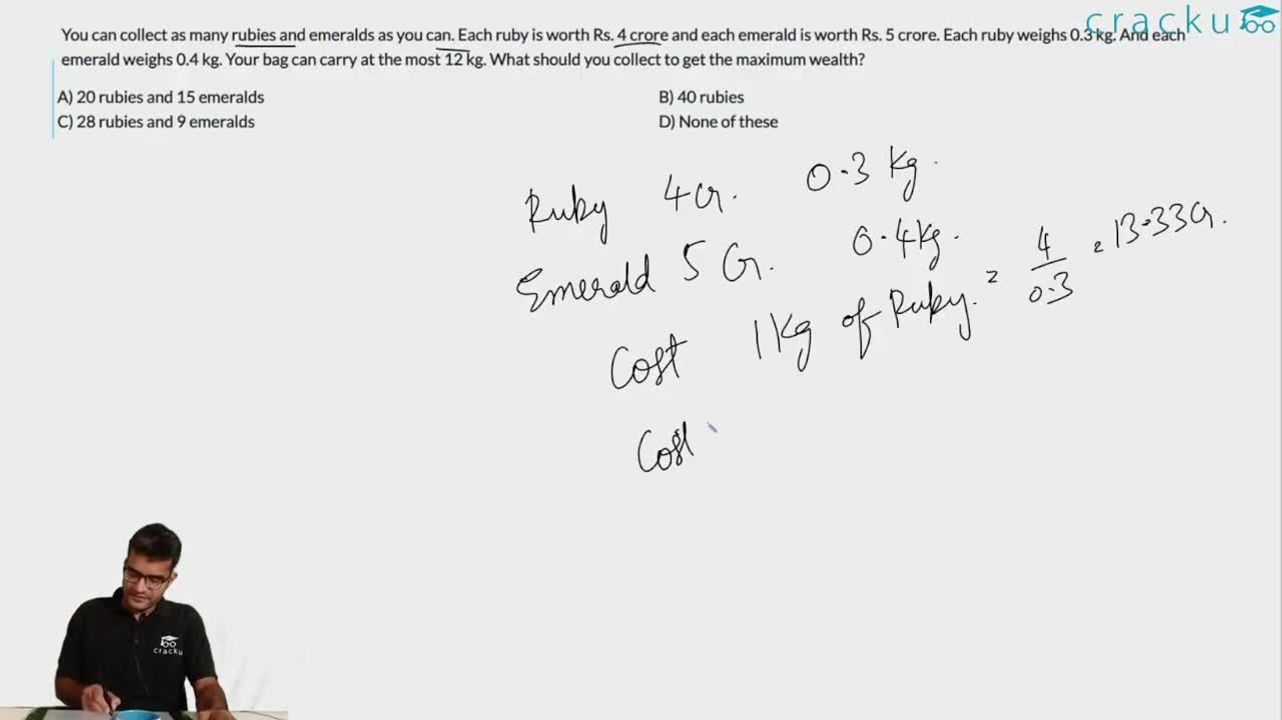Question 71

# You can collect as many rubies and emeralds as you can. Each ruby is worth Rs. 4 crore and each emerald is worth Rs. 5 crore. Each ruby weighs 0.3 kg. And each emerald weighs 0.4 kg. Your bag can carry at the most 12 kg. What should you collect to get the maximum wealth?

Solution

Let's say number of rubbies are x and emeralds are y.
So 0.3x + 0.4y = 12
And total wealth = 4x+5y
Now putting value of x from eq.1 to eq.2
i.e. total wealth = 4(12-0.4y)/0.3 + 5y
Now for maximizing total wealth y should be equal to zero.
Hence x = 40

### View Video Solution